## Sunday, July 4, 2010

### Multiple length cutting stock problem

I received some test data to run against a cutting stock model (see http://yetanothermathprogrammingconsultant.blogspot.com/2009/09/vstosolver-foundation.html). The data approximately looks like: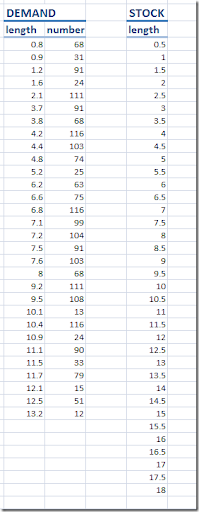This cannot be solved using the standard model mentioned here and in http://www.amsterdamoptimization.com/pdf/colgen.pdf. The standard model assumes there is just one raw material length, and we have here a number of different lengths in stock. Note that we assume that stock availability is not a limiting factor. The question was: can we adapt the column generation approach to handle this.
The first document I found with google was this: http://www.aimms.com/aimms/download/manuals/aimms3om_cuttingstock.pdf. At first reading this did not look very promising for different reasons. First they use as objective in the multiple length version: minimize the cost of each stock length. I don’t have this data available, and we are aiming to minimize waste.  Note that in the standard cutting stock problem we can simply use the number of rolls of raw material as proxy to find the minimum waste. In this case this is not a good objective and we really need to model the wasted material explicitly.
A second problem was the simple way they dealt with the multiple lengths in the sub problem. They just run the subproblem several times: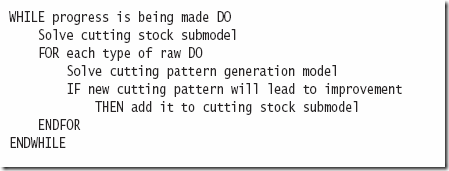In our case we have a somewhat large set of stock lengths, so that is not attractive. It was argued one could stop as soon as a good candidate was found. (Note: There is some confusion about what the sub and the master is as they use the term submodel for what usually is called the master problem.)
I believe there is a more elegant way to do this: let the pattern generation model (this is usually called the subproblem) find a candidate pattern and a candidate raw material length directly. A similar approach is written up here.
So with the notation: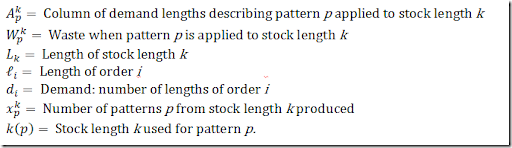we have:and we can form a master problem as follows: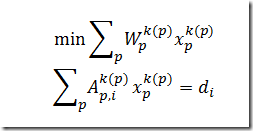Note that I used an equality constraint here. That is to make sure we don’t produce more products with zero waste than needed. The subproblem can now look like: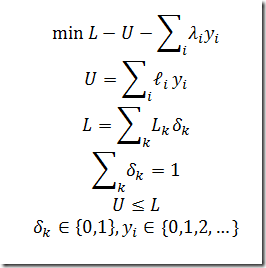Here lambda are the duals of the master problem. So the subproblem both finds a new pattern y(i) and which stock length k is to be used for this pattern. This actually solved the problem quickly (approx 10 seconds). A further improvement was added by preprocessing the data. If a demand length is equal to a stock length, we can immediately choose that stock length, and remove this demand from the model. If the problem is larger, it may be wise to add a tolerance, i.e. if a demanded length is within say 5% of a stock length, use that stock length.
It is interesting to see that the column generation algorithm actually is not that sensitive to the preprocessing step: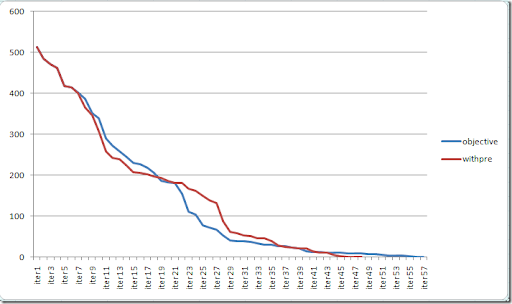We stop a little bit earlier with preprocessing, but the expected better performance is not uniformly over the whole run. It is noted I used a simple minded assignment for the initial solution. Probably a simple bin-packing heuristic to find an initial solution can help to start the column generation algorithm with a better objective.
An alternative formulation would be: use inequality constraint in the master together with a cost coefficient of c(p)=L(k(p)). The subproblem needs to be updated slightly: drop U from the objective. This formulation gave similar results.

The solution looks like:

1.2.3.